# 神经网络基础部件-激活函数详解

+关注继续查看

## 一，激活函数概述

### 1.1，前言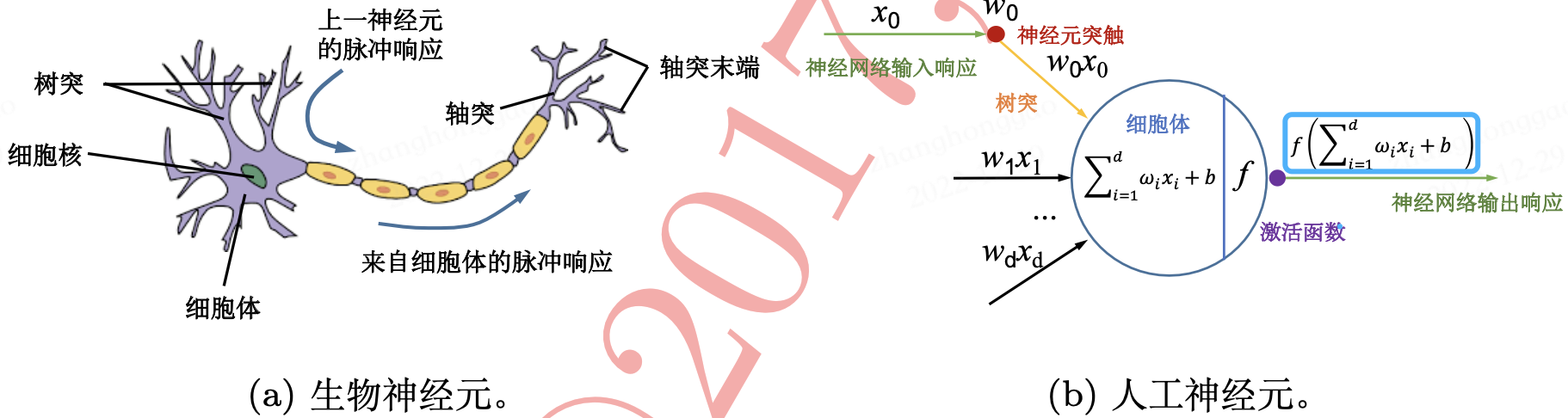### 1.2，激活函数定义

$$z = \sum_{d=1}^{D} w_{d}x_{d} + b = w^\top x + b$$

$$a = f(z)$$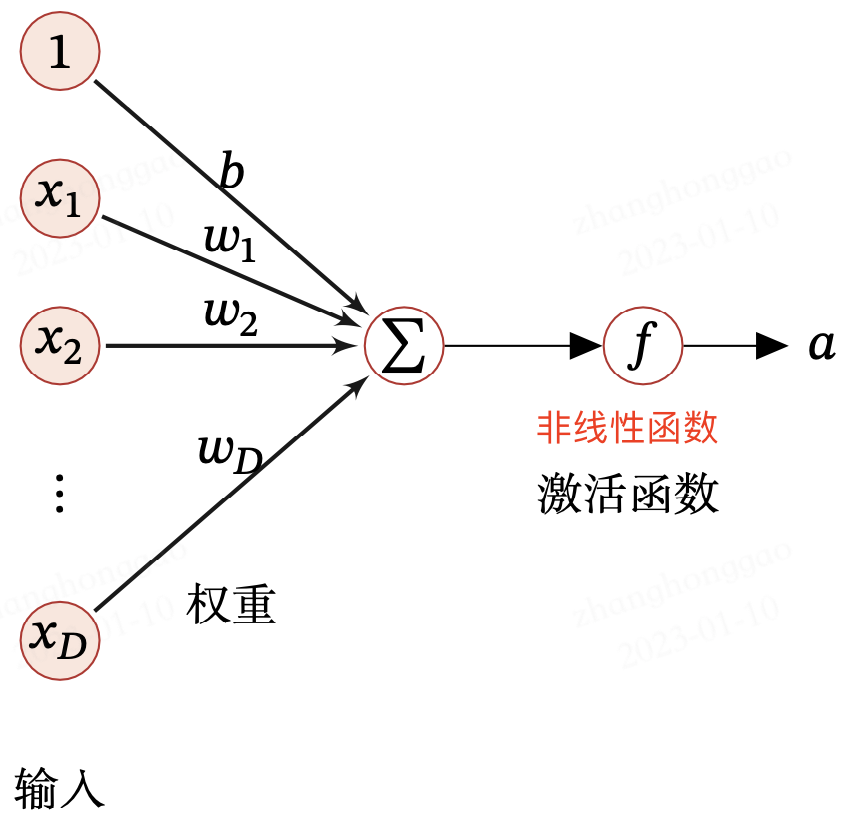### 1.3，激活函数性质

1. 连续并可导(允许少数点上不可导)的非线性函数。可导的激活函数 可以直接利用数值优化的方法来学习网络参数。
2. 激活函数及其导函数要尽可能的简单，有利于提高网络计算效率。
3. 激活函数的导函数的值域要在一个合适的区间内，不能太大也不能太小，否则会影响训练的效率和稳定性.

## 二，Sigmoid 型函数（挤压型激活函数）

Sigmoid 型函数是指一类 S 型曲线函数，为两端饱和函数。常用的 Sigmoid 型函数有 Logistic 函数和 Tanh 函数。

### 2.1，Logistic(sigmoid)函数

$$\sigma(x) = \frac{1}{1 + exp(-x)}$$

sigmoid 函数的导数公式如下所示:

$$\frac{\mathrm{d} }{\mathrm{d} x}\text{sigmoid}(x) = \frac{exp(-x)}{(1+exp(-x))^2} = \text{sigmoid}(x)(1 - \text{sigmoid}(x))$$

sigmoid 函数及其导数曲线如下所示: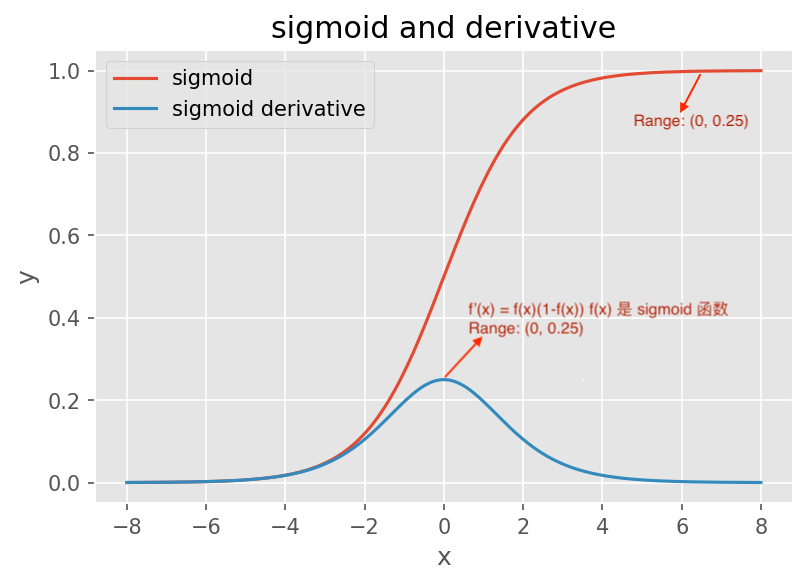nn.BCEWithLogitsLoss() 函数等效于 sigmoid + nn.BCELoss

### 2.2，Tanh 函数

Tanh（双曲正切）函数也是一种 Sigmoid 型函数，可以看作放大并平移的 Sigmoid 函数，公式如下所示：

$$\text{tanh}(x) = 2\sigma(2x) - 1 = \frac{2}{1 + e^{-2x}} - 1$$

$$\frac{\mathrm{d} }{\mathrm{d} x} \text{tanh}(x) = 1 - \text{tanh}^{2}(x)$$

Logistic 和 Tanh 两种激活函数的实现及可视化代码（复制可直接运行）如下所示:

# example plot for the sigmoid activation function
import numpy as np
from matplotlib import pyplot
import matplotlib.pyplot as plt

# sigmoid activation function
def sigmoid(x):
"""1.0 / (1.0 + exp(-x))
"""
return 1.0 / (1.0 + np.exp(-x))

def tanh(x):
"""2 * sigmoid(2*x) - 1
(e^x – e^-x) / (e^x + e^-x)
"""
# return (exp(x) - exp(-x)) / (exp(x) + exp(-x))
return 2 * sigmoid(2*x) - 1

def relu(x):
return max(0.0, x)

"""1 * (x > 0)"""
if x < 0.0:
return 0
else:
return 1

"""sigmoid(x)(1−sigmoid(x))
"""
a = sigmoid(x)
b = 1 - a
return a*b

return 1 - tanh(x)**2

# 1, define input data
inputs = [x for x in range(-6, 7)]

# 2, calculate outputs
outputs = [sigmoid(x) for x in inputs]
outputs2 = [tanh(x) for x in inputs]

# 3, plot sigmoid and tanh function curve
plt.figure(dpi=100) # dpi 设置
plt.style.use('ggplot') # 主题设置

plt.plot(inputs, outputs, label='sigmoid')
plt.plot(inputs, outputs2, label='tanh')

plt.xlabel("x") # 设置 x 轴标签
plt.ylabel("y")
plt.title('sigmoid and tanh') # 折线图标题
plt.legend()
plt.show()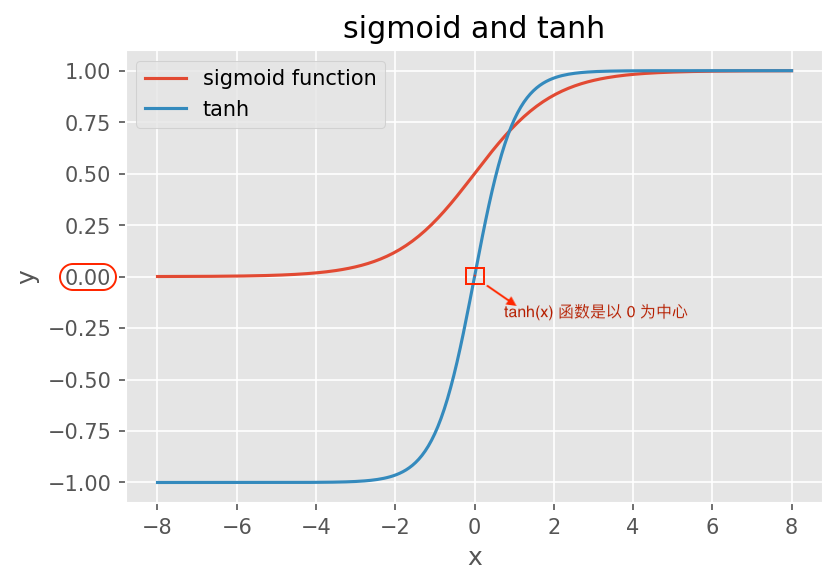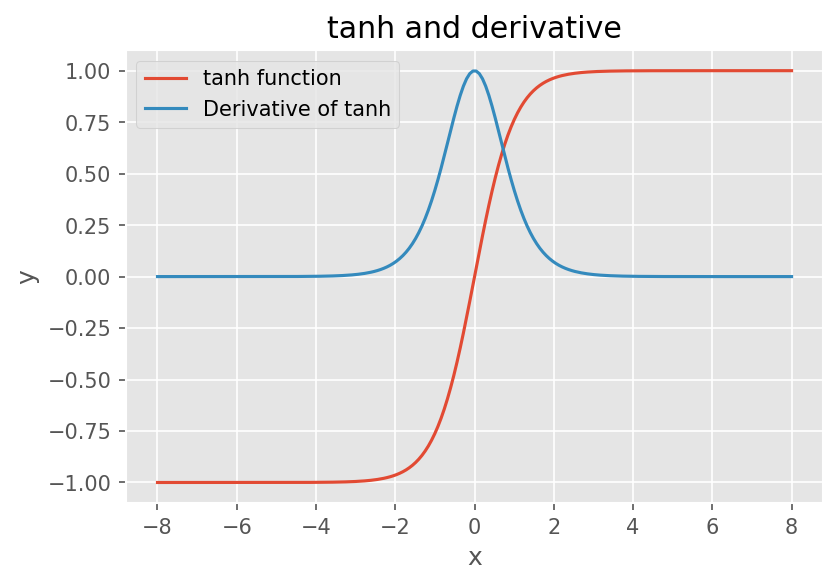Tanh 函数优缺点总结

• 具有 Sigmoid 的所有优点。
• exp 指数计算代价大。梯度消失问题仍然存在。

Tanh 函数及其导数曲线如下所示:

Tanh 和 Logistic 函数的导数很类似，都有以下特点:

• 当输入接近 0 时，导数接近最大值 1。
• 输入在任一方向上越远离0点，导数越接近0。

## 三，ReLU 函数及其变体（半线性激活函数）

### 3.1，ReLU 函数

ReLU(Rectified Linear Unit，修正线性单元)，是目前深度神经网络中最经常使用的激活函数，它保留了类似 step 那样的生物学神经元机制: 输入超过阈值才会激发。公式如下所示:

$$ReLU(x) = max(0, x) = \left \lbrace \begin{matrix} x & x\geq 0 \\ 0 & x< 0 \end{matrix}\right.$$

1，优点:

• ReLU 激活函数计算简单
• 具有很好的稀疏性，大约 50% 的神经元会处于激活状态。
• 函数在 x > 0 时导数为 1 的性质（左饱和函数），在一定程度上缓解了神经网络的梯度消失问题，加速梯度下降的收敛速度。

2，缺点:

• ReLU 函数的输出是非零中心化的，给后一层的神经网络引入偏置偏移，会影响梯度下降的效率
• ReLU 神经元在训练时比较容易“死亡”。如果神经元参数值在一次不恰当的更新后，其值小于 0，那么这个神经元自身参数的梯度永远都会是 0，在以后的训练过程中永远不能被激活，这种现象被称作“死区”。

ReLU 激活函数的代码定义如下:

# pytorch 框架对应函数： nn.ReLU(inplace=True)
class ReLU(object):

def func(self, x):
return np.maximum(x, 0.0)

def derivative(self, x):
"""简单写法: return x > 0.0"""
da = np.array([1 if x > 0 else 0 for x in a])
return da     

ReLU 激活函数及其函数梯度图如下所示: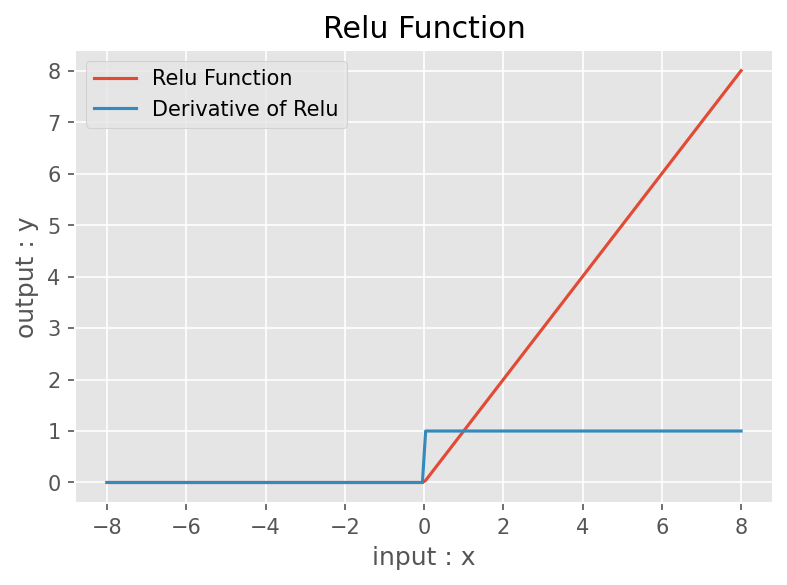ReLU 激活函数的更多内容，请参考原论文 Rectified Linear Units Improve Restricted Boltzmann Machines

### 3.2，Leaky ReLU/PReLU/ELU/Softplus 函数

1，Leaky ReLU 函数: 为了缓解“死区”现象，研究者将 ReLU 函数中 x < 0 的部分调整为 $\gamma \cdot x$， 其中 $\gamma$ 常设置为 0.01 或 0.001 数量级的较小正数。这种新型的激活函数被称作带泄露的 ReLULeaky ReLU）。

$$\text{Leaky ReLU}(x) = max(0, 𝑥) + \gamma\ min(0, x) = \left \lbrace \begin{matrix} x & x\geq 0 \\ \gamma \cdot x & x< 0 \end{matrix}\right.$$

2，PReLU 函数: 为了解决 Leaky ReLU 中超参数 $\gamma$ 不易设定的问题，有研究者提出了参数化 ReLU(Parametric ReLU，PReLU)。参数化 ReLU 直接将 $\gamma$ 也作为一个网络中可学习的变量融入模型的整体训练过程。对于第 $i$ 个神经元，PReLU 的 定义为:

$$\text{Leaky ReLU}(x) = max(0, 𝑥) + \gamma_{i}\ min(0, x) = \left\lbrace\begin{matrix} x & x\geq 0 \\ \gamma_{i} \cdot x & x< 0 \end{matrix}\right.$$

3，ELU 函数: 2016 年，Clevert 等人提出的 ELU (Exponential Linear Units) 在小于零的部分采用了负指数形式。ELU 有很多优点，一方面作为非饱和激活函数，它在所有点上都是连续的和可微的，所以不会遇到梯度爆炸或消失的问题；另一方面，与其他线性非饱和激活函数（如 ReLU 及其变体）相比，它有着更快的训练时间和更高的准确性。

$$\text{Leaky ReLU}(x) = max(0, 𝑥) + min(0, \gamma(exp(x) - 1) = \left\lbrace\begin{matrix} x & x\geq 0 \\ \gamma(exp(x) - 1) & x< 0 \end{matrix}\right.$$

$\gamma ≥ 0$ 是一个超参数，决定 $x ≤ 0$ 时的饱和曲线，并调整输出均值在 0 附近。

4，Softplus 函数: Softplus 函数其导数刚好是 Logistic 函数.Softplus 函数虽然也具有单侧抑制、宽 兴奋边界的特性，却没有稀疏激活性。Softplus 定义为:

$$\text{Softplus}(x) = log(1 + exp(x))$$

Softplus 有兴趣的可以阅读这篇论文: 《Deep Sparse Rectifier Neural Networks》

ReLU、Leaky ReLU、ELU 以及 Softplus 函数示意图如下图所示: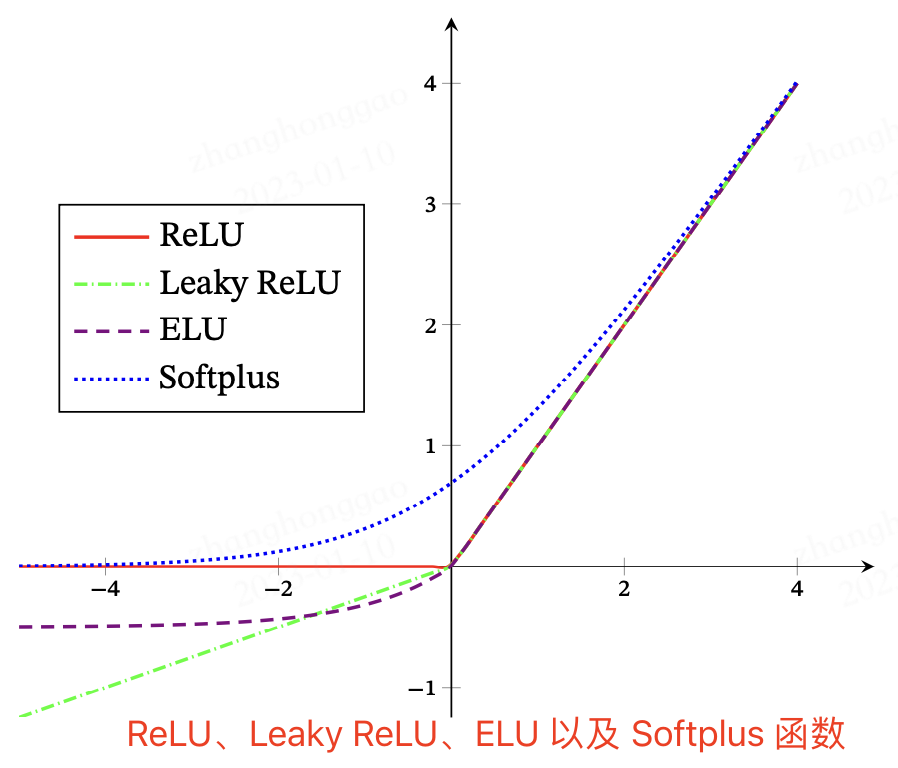## 四，Swish 函数

Swish 函数[Ramachandran et al., 2017] 是一种自门控(Self-Gated)激活 函数，定义为

$$\text{swish}(x) = x\sigma(\beta x)$$

Swish 函数代码定义如下：

# Swish https://arxiv.org/pdf/1905.02244.pdf
class Swish(nn.Module):  #Swish激活函数
@staticmethod
def forward(x, beta = 1): # 此处beta默认定为1
return x * torch.sigmoid(beta*x)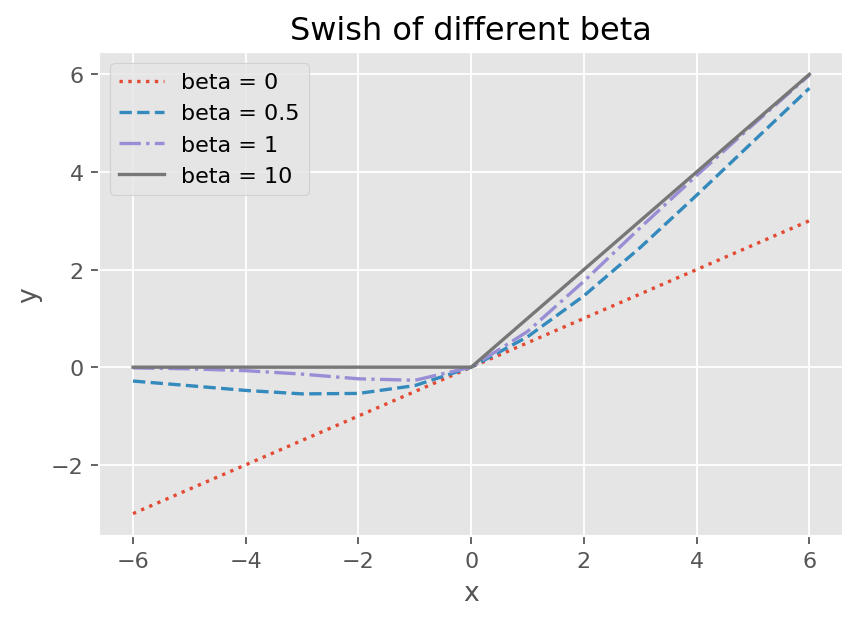Swish 函数可以看作线性函数和 ReLU 函数之间的非线性插值函数，其程度由参数 $\beta$ 控制

## 五，激活函数总结

from math import exp

class Sigmoid(object):

def func(self, x):
return 1.0 / (1.0 + np.exp(-x))

def derivative(self, x):
return self.func(x) * (1.0 - self.func(x))

class Tanh(object):

def func(self, x):
return np.tanh(x)

def derivative(self, x):
return 1.0 - self.func(x) ** 2

class ReLU(object):

def func(self, x):
return np.maximum(x, 0.0)

def derivative(self, x):
return x > 0.0

class LeakyReLU(object):

def __init__(self, alpha=0.2):
super().__init__()
self.alpha = alpha

def func(self, x):
return np.array([x if x > 0 else self.alpha * x for x in z])

def derivative(self, x):
dx = np.array([1 if x > 0 else self.alpha for x in a])
return dx

class Softplus(object):

def func(self, x):
return np.log(1 + np.exp(z))

def derivative(self, x):
return 1.0 / (1.0 + np.exp(-x))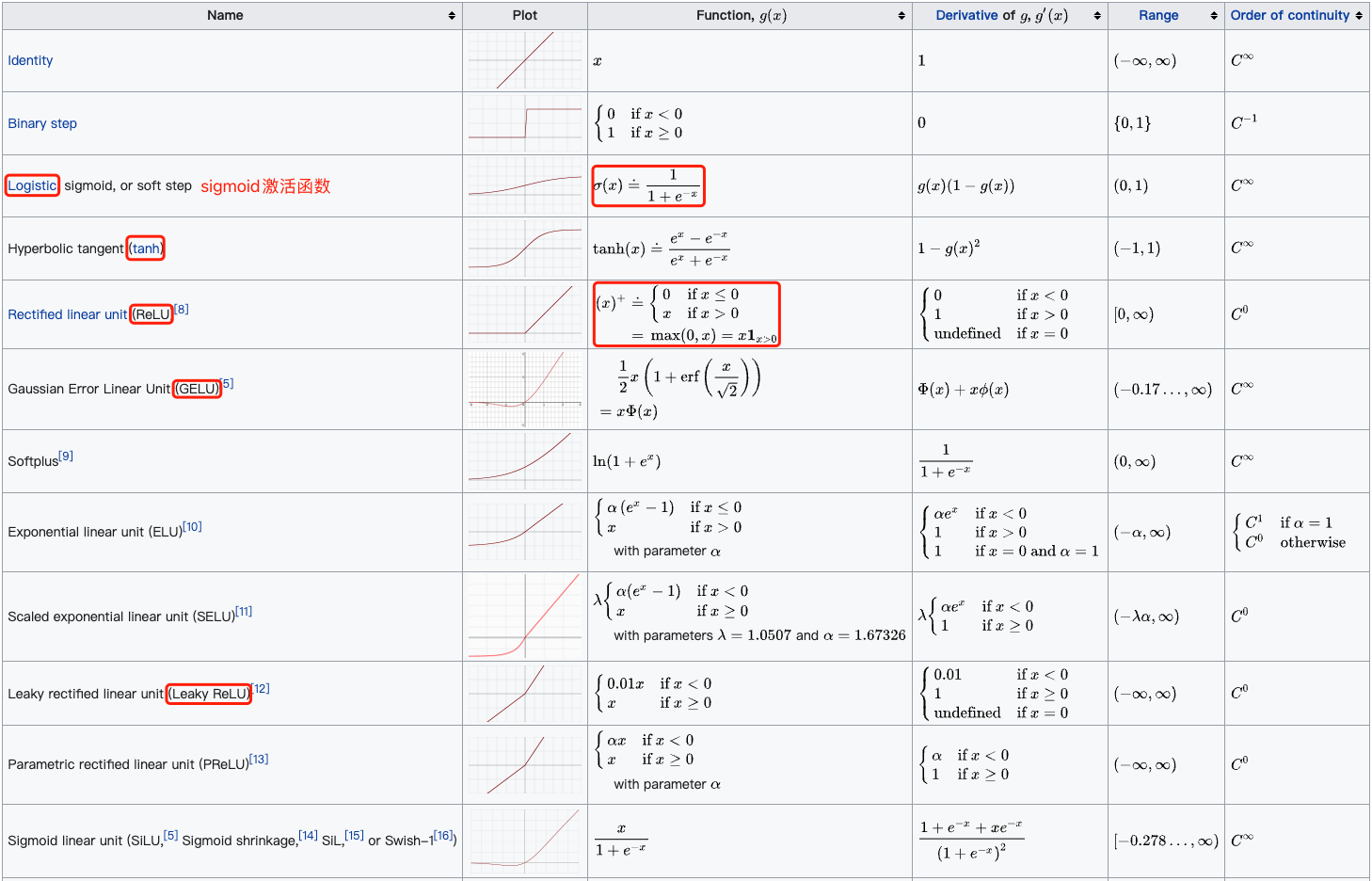## 参考资料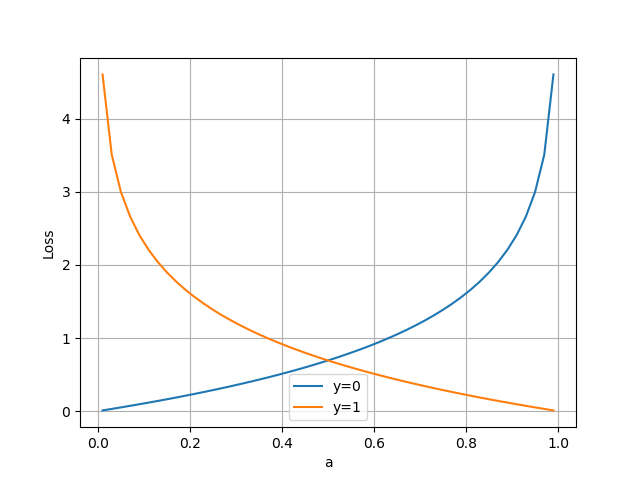50 020 0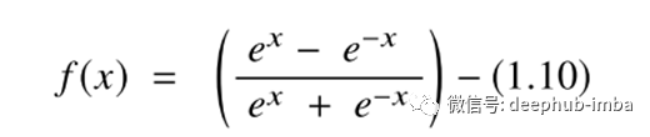5分钟了解神经网络激活函数（二）
5分钟了解神经网络激活函数（二）
47 05分钟了解神经网络激活函数（三）
5分钟了解神经网络激活函数（三）
31 0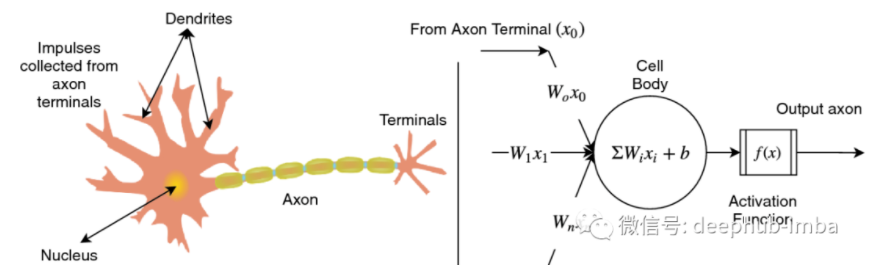5分钟了解神经网络激活函数（一）
5分钟了解神经网络激活函数（一）
61 0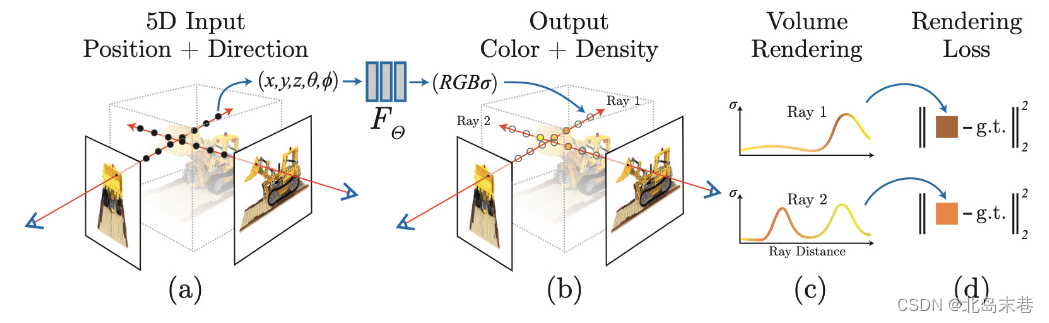NeRF神经网络介绍

311 0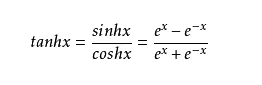83 0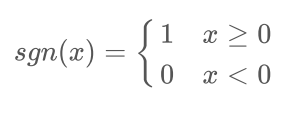88 066 0

🏆 阿里云社区专家博主，大厂算法开发工程师。 💻 从事视觉算法开发和模型压缩部署工作。 👍 欢迎关注我的公众号-嵌入式视觉。7552757636

【算法实战】5. Logistic回归算法396

【算法实战】9. 线性回归算法315

【算法实战】6. 支持向量机算法5310

【算法实战】2. K近邻算法1325